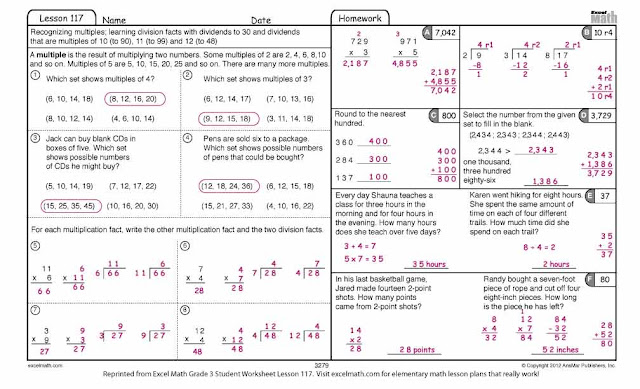## Wednesday, May 23, 2012

### Math Multiples & Division Worksheet

multiple is the result of multiplying two numbers. Some multiples of 2 are:
2, 4, 6, 8, 10 and so on

Some multiples of 5 are 5, 10, 15, 20, 25, and so on.

In Excel Math, we teach students how to multiply and divide, how to find the greatest common factor between two or more numbers, how to identify fact families, and how to recognize multiples.

Help your students identify multiples by writing the first three multiples of 2 on the board. Let your students list two additional multiples of 2. Do the same with multiples of 5. If you have time, continue by listing multiples of 3 and 4. Then have your students complete problems 1-4 on this Grade 3 Excel Math worksheet. Do the first one together:Excel Math Grade 3 Student worksheet Click here to download a PDF file of this worksheet

Before they begin number 2, remind the students that 3 will divide evenly into any number whose digits add up to a multiple of 3. So 3 will divide evenly into 189 (the digits of 189 add up to 18, which is evenly divisible by 3). Three will not divide evenly into 17 (the digits of 17 add up to 8, which is not evenly divisible by 3). Let the students finish #2-4 on their own. The answers are shown below.

Arrange students in pairs. Give each student 33 items that can be used for counting (paper clips, beans, pennies, etc.) Point out that factors are all the integers that divide evenly into another integer (whole number). They are "what you get" when you divide. The number always works as a factor.

Read the instructions for number 5. Ask the students to tell you which numbers are the factors in the problem. (11 and 6) Have the students use their counters to form 6 groups with 11 in each  group. Let them count the total items and print the answer under the line. (66)

Next, have them take the 66 items and see how many times they can subtract six. Each time they subtract six, ask them what the result is. (The result will be multiples of 6.)

Ask the students how many times they were able to subtract 6. To reinforce the connection between division and subtraction, write on the board: There are 11 groups of 6 in 66.

Explain that another multiplication fact in this fact family would be to simply rearrange the order of the factors: 11 x 6. Have the students write 6 x 11 above the empty line. Have them form 11 groups of 6 with their items. Let them count the total items and print the answer under the line. (66)

Now have the students take the 66 items and see how many times they can subtract eleven. Each time they subtract 11, ask them what the result is. (The result will be multiples of 11.)

Repeat this process for numbers 6-8. Point out that multiplication and division problems form fact families just as addition and subtraction do. Here are the answers:Excel Math Grade 3 Lesson 117 Student Worksheet with Answers Click here to download a PDF file of this worksheet
Since Excel Math uses a unique spiraling strategy, you will need to teach multiple lessons sequentially within each grade level in order to get the concepts into your students' long-term memory. A student's learning of new concepts takes place during Excel Math Lesson Plans and Activities. The concepts are refreshed through Guided Practice, Homework, Create A Problem and Tests.

Visit Excel Math online to find out more about our lesson plans and student worksheets. Excel Math is available for Kindergarten through Grade 6.